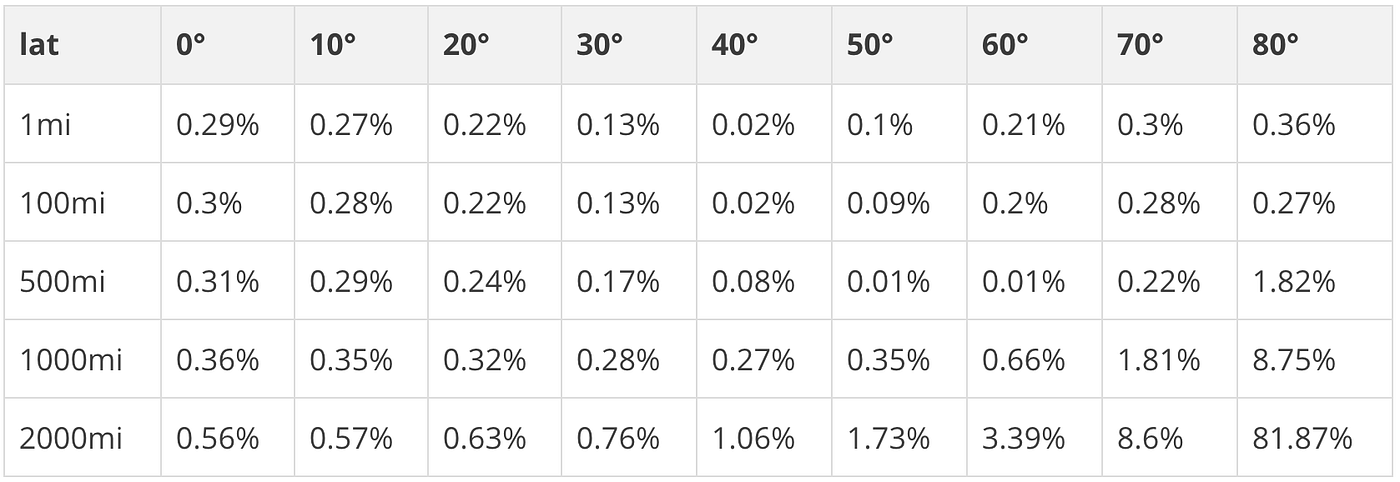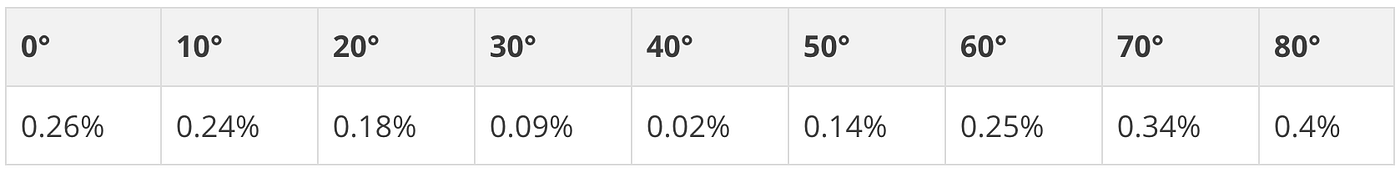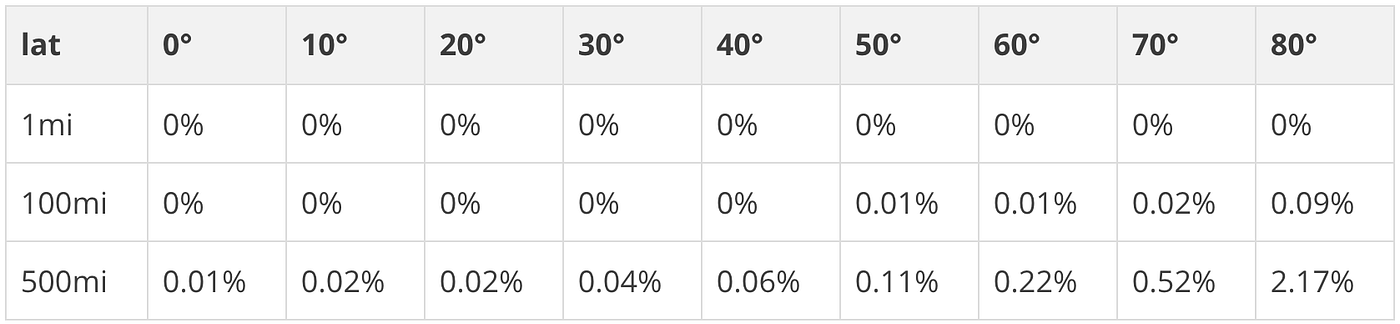# 使用「Cheap Ruler」进行快速测地线逼近

Posted on Mar 27, 2022

• 在对精度不是很敏感的场景上，用地球是半径 6371 公里的球的假设也足够用；
• 精度要求高，计算量会显著增加，比如文森特公式；
• Mapbox 开源的 cheapruler 库在绝大多数城市级别（纬度相近）地理计算上比传统的计算方法效率高得多，并且误差在可接受的范围上。 实现上非常讨巧，在部分地理计算是瓶颈的场景下能显著提升处理速度；

## 欧几里德测地线近似

$$\partial y=12430 \frac{\left|lat_{1}-lat_{2}\right|}{180}$$

$$\partial x =24901 \frac{\left|lng_{1}-lng_{2}\right|}{360} \cos(lat)$$

\begin{aligned} \partial y &=12430 \frac{\left|lat_{1}-lat_{2}\right|}{180} \newline \partial x &=24901 \frac{\left|lng_{1}-lng_{2}\right|}{360} \cos \left(\frac{lat_{1}+lat_{2}}{2}\right) \newline d &=\sqrt{\partial x^{2}+\partial y^{2}} \end{aligned}

## 提高近似精度\begin{aligned} K_{1}&=111.13209-0.56605 \cos(2lat)+0.0012 \cos(4lat) \newline K_{2}&=111.41513 \cos (lat)-0.09455 \cos (3lat)+0.00012 \cos (5lat) \newline d&=\sqrt{\left(K_{1}\left(lat_{1}-lat_{2}\right)\right)^{2}+\left(K_{2}\left(lng_{1}-lng_{2}\right)\right)^{2}} \end{aligned}## 介绍 Cheap Ruler

• distance: 快了 31 倍
• bearing: 快了 3.6 倍
• destination: 快了 7.2 倍
• lineDistance: 快了 31 倍
• area: 快了 3.4 倍
• along: 快了 31 倍
• pointOnLine: 快了 78 倍
• lineSlice: 快了 60 倍

• lineSliceAlong: 在给定的距离之间沿线划一条线，比 turf.lineSlice(turf.along(... 快 285 倍
• bufferPoint: 给定一个缓冲区距离，在一个点周围创建一个边界框; 比用 turf.destination 创建两个对角线的边界框快 260 倍
• bufferBBox: 快 260 倍
• insideBBox: 比 turf.inside(turf.point(p), turf.bboxPolygon(bbox)) 快 19 倍

var ruler = cheapRuler(35.05, "miles");


var ruler = cheapRuler.fromTile(1567, 12, "kilometers");


var distance = ruler.distance([30.51, 50.32], [30.52, 50.312]);